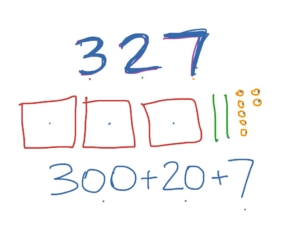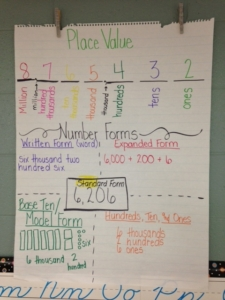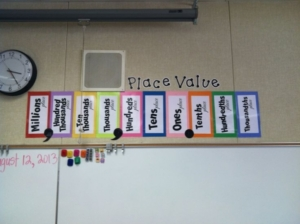What is Place Value?
Place Value is the value of a digit in a number based on the location of the digit. Place Value understanding is essential for students to add and subtract multi-digit numbers. Students need to understand that the place value system we use is in base ten is comprised of ones, tens, hundreds and thousands.

In 3rd grade, students are expected to:
• Use place value understanding to round whole numbers to the nearest 10 or 100.
• Fluently add and subtract within 1,000, using strategies and algorithms based on place value.
• Multiply one-digit whole numbers by multiples of 10 in the range of 10-90 using strategies based on place value.

How can we strengthen students’ understanding of place value?
Using place value to represent numbers is an important skill that students will need in order to add and subtract using place value understanding and vocabulary.

Here are different ways to explore and represent numbers ruing place value:
1. Base ten models – Students are given tasks to explore and understand representing numbers with base ten blocks and later move to drawing quick picture representations of these numbers. This helps them understand what a number is comprised of. A place value chart can be utilized with these models.

Typically the exploration with these tools begins by handing a unit (ones), rod (tens), flat (hundreds), and a cube (thousands) and posing the question, “How do these tools relate?” Through exploration, you want students to realize that it takes ten units to make a rod (ten ones to make a ten), ten rods make a flat (ten tens make a hundred) and ten flats make a cube (ten hundreds make a thousand).2. Expanded Form – Representing numbers based on the value of each digit in a number. For example 417 in expanded form is 400 + 10 + 7 based on the location of each digit. You want to continue making this process hands-on by using number stack-up cards.3. Base ten language – Using place value language to identify what each digit’s value is. For example 582 is worth 5 hundreds, 8 tens and 2 ones.

4. Word Form – Important to have students use and understand place value vocabulary. Record numbers in word form exactly as you say them out-loud, such as 936 as “nine hundred thirty-six.”

5. It could also help to create an anchor chart with the different representations and place value language. Keep referring students to the anchor chart when discussing, writing and representing numbers with place value language.6. Representing numbers flexibly is another essential strategy students learned in 2nd grade, which help with developing a deeper understanding of number and place value. For example: 316 can be represented as 3 hundreds, 1 ten and 6 ones OR 2 hundreds, 11 tens and 6 ones. This is not a specified strategy in the 3rd grade standards, but you may want to keep it in mind as students are representing numbers and applying invented strategies when adding and subtracting.

When should we be building place value understanding?
The Building Math Community during the first 9 days is a great time to reinforce place value concepts students learned in 2nd grade so they will be ready to apply the understanding of number to addition/subtraction concepts. Place Value centers and morning work are also great opportunities to spiral review and reinforce these place value concepts and continue to connect to upcoming units/concepts.

Make sure to post and make visible the place value language and models you want your students to utilize while building understanding of place value.For great free printable resources to explore expanded form in a hands-on exploration with stackable number cards, visit the website http://goo.gl/9112KJ.

Another great resource for composing and decomposing numbers into expanded form or standard form is https://illuminations.nctm.org/Lesson.aspx?id=3691 from NCTM Illuminations.